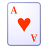# New to Qlik Sense

If you’re new to Qlik Sense, start with this Discussion Board and get up-to-speed quickly.

Announcements
Become an analytics expert with Qlik's new 15 week course: Applied Data Analytics using Qlik Sense. READ MORE
cancel
Showing results for
Did you mean:Contributor III

## Adding Pivot Table Total in Qlik Sense

My Conversion Rate Calc is as follows:

Sum(Transactions)/Sum(Average Visitors)

I have the below conditions that state if Conversiion Rate Calc is null then null and if its between 10% and 60% then return the Conversion Rate else make it zero

If(IsNull(Sum(Transactions)/Sum([Average visitors])), 0,
If((Sum(Transactions)/Sum([Average visitors]))>0.6, 0,
If((Sum(Transactions)/Sum([Average visitors]))>0.1, (Sum(Transactions)/Sum([Average visitors])))))

The result is correct for each store but the total is not correct

1018665/3060186=33,3% and not 26563,8%

I've tried this expression but it's not working

Sum(Aggr((If(IsNull(Sum(Transactions)/Sum([Average visitors])), 0,
If((Sum(Transactions)/Sum([Average visitors]))>0.6, 0,
If((Sum(Transactions)/Sum([Average visitors]))>0.1, (Sum(Transactions)/Sum([Average visitors])),0)))), Store, Date))

This works for the rows but it's not giving the correct total.

Labels (15)

• ### Set Analysis Modifiers

1 Solution

Accepted SolutionsMVP

Hi, instead of a sum of the percentages you need a sum of each of the values separatedly and then calcualte the percentage, something like:

``````Sum(Aggr((If(IsNull(Sum(Transactions)/Sum([Average visitors])), 0,
If((Sum(Transactions)/Sum([Average visitors]))>0.6, 0,
If((Sum(Transactions)/Sum([Average visitors]))>0.1, (Sum(Transactions)),0)))), Store, Date))
/
Sum(Aggr((If(IsNull(Sum(Transactions)/Sum([Average visitors])), 0,
If((Sum(Transactions)/Sum([Average visitors]))>0.6, 0,
If((Sum(Transactions)/Sum([Average visitors]))>0.1, (Sum([Average visitors])),0)))), Store, Date))``````

If in script you flag records by Store and date where they accomplish that Sum(Transactions)/Sum([Average visitors])>0.1 and <0.6, you will only need:

``Sum({<flagField={1}>} Transactions)/Sum({<flagField={1}>} [Average visitors])``

And this will work for rows and for totals, with better performance than using aggr.

5 RepliesMVP

Hi, in this case you don't have to use aggr because it will sum each percentage. I don't see why the first expression will return that high %, it should work using the same expression as by store. Is that result with just the first expression or with the aggr applied?

Other expression may be needed if youwant to exclude some stores in the total rowContributor III
Author

Hi, I need the second expression to work as I am excluding some stores in the total. The second expression is giving me the high%MVP

Can you please share the sample app?

Thanks & Regards,
Mayil Vahanan RMVP

Hi, instead of a sum of the percentages you need a sum of each of the values separatedly and then calcualte the percentage, something like:

``````Sum(Aggr((If(IsNull(Sum(Transactions)/Sum([Average visitors])), 0,
If((Sum(Transactions)/Sum([Average visitors]))>0.6, 0,
If((Sum(Transactions)/Sum([Average visitors]))>0.1, (Sum(Transactions)),0)))), Store, Date))
/
Sum(Aggr((If(IsNull(Sum(Transactions)/Sum([Average visitors])), 0,
If((Sum(Transactions)/Sum([Average visitors]))>0.6, 0,
If((Sum(Transactions)/Sum([Average visitors]))>0.1, (Sum([Average visitors])),0)))), Store, Date))``````

If in script you flag records by Store and date where they accomplish that Sum(Transactions)/Sum([Average visitors])>0.1 and <0.6, you will only need:

``Sum({<flagField={1}>} Transactions)/Sum({<flagField={1}>} [Average visitors])``

And this will work for rows and for totals, with better performance than using aggr.Contributor III
Author

This worked perfectly, thank you.